# 数据库中的 B 树与 B+ 树

## 学习索引笔记

Posted by YEY on January 2, 2021

# 数据库中的 B 树与 B+ 树

## 1. 主要内容

B 树与 B+ 树是一类非常重要的数据结构，它们被广泛应用于各种数据库中。为了更好地理解这类数据结构，我们将逐一讨论以下内容：

1. 磁盘结构
2. 数据是如何存储在磁盘上的
3. 什么是索引
4. 什么是多级索引
5. 多路查找树
6. B 树
7. B 树中的插入与删除
8. B+

## 2. 磁盘结构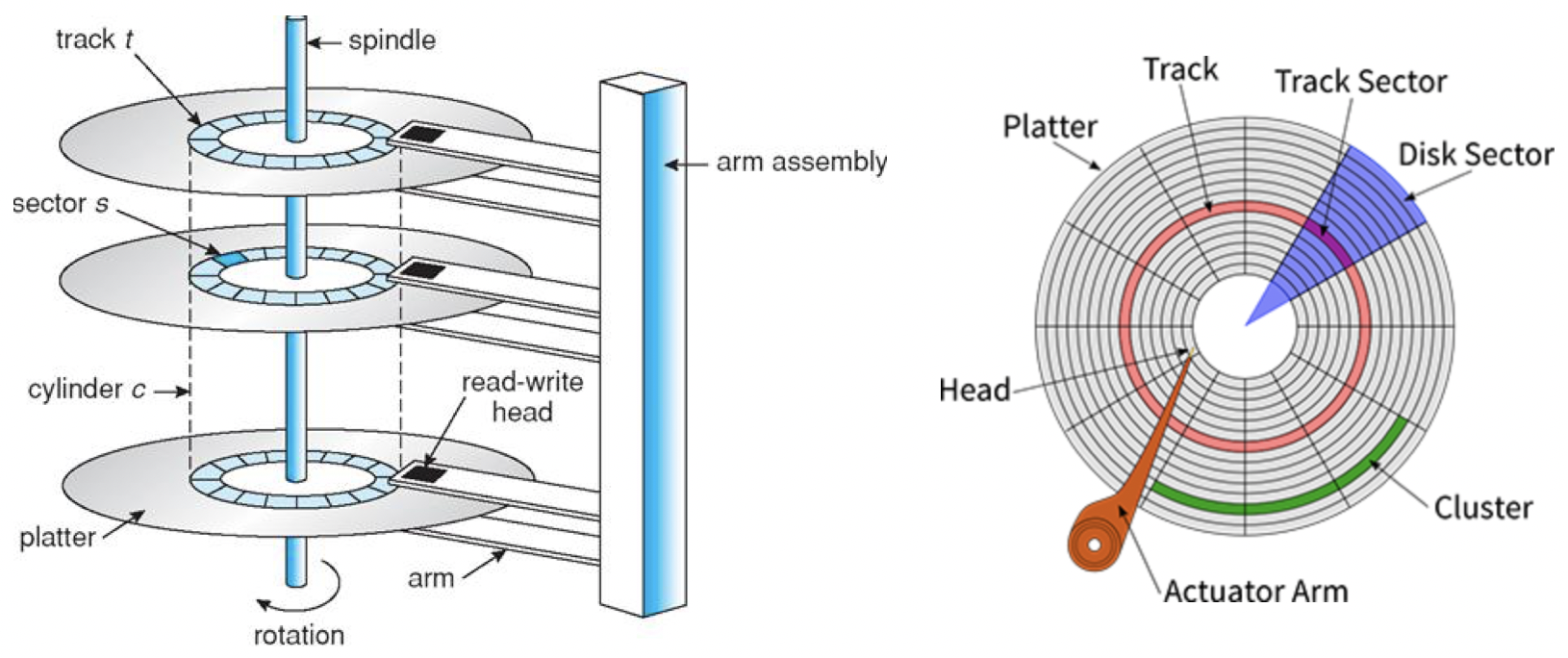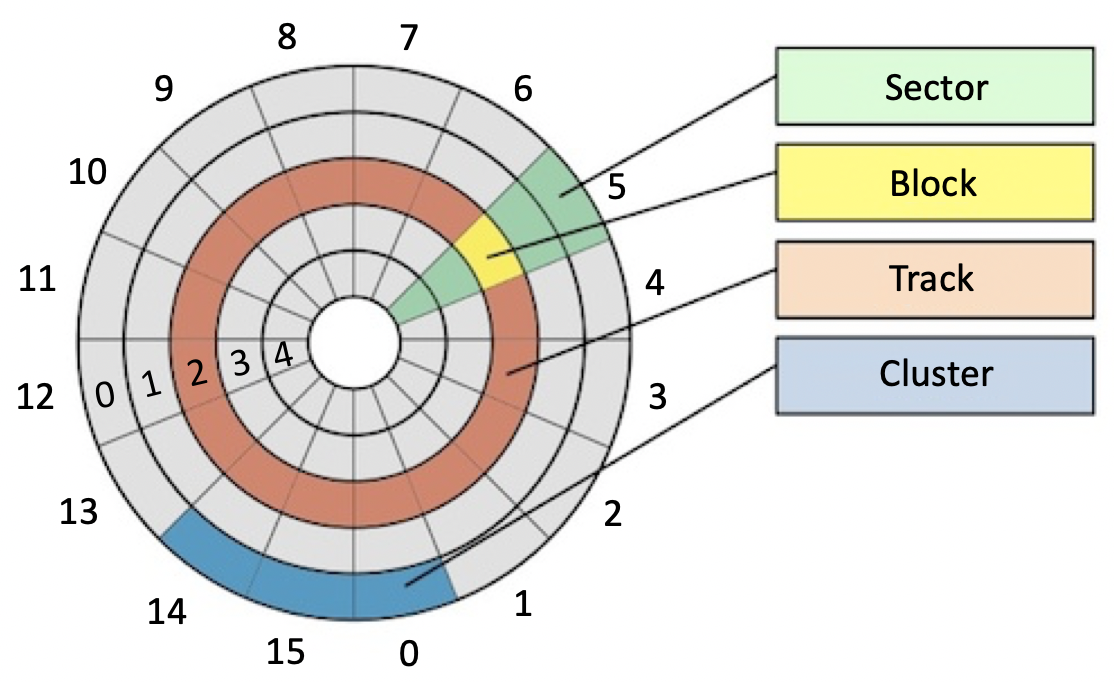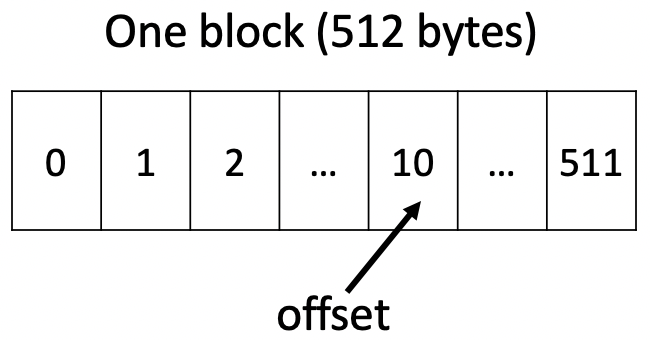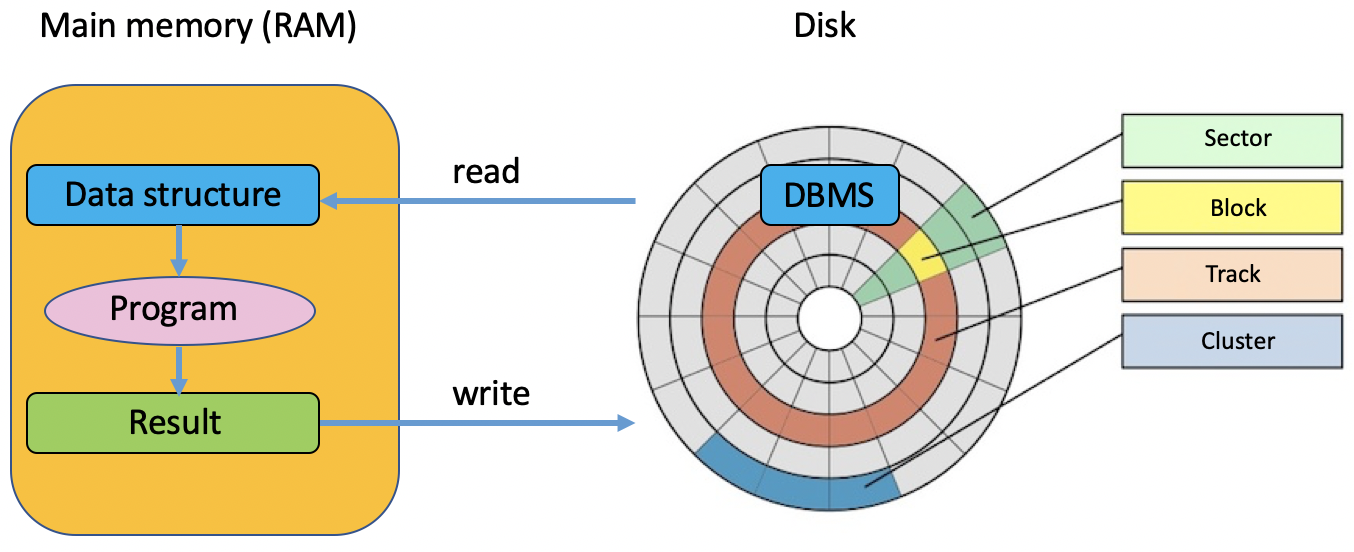## 3. 数据是如何存储在磁盘上的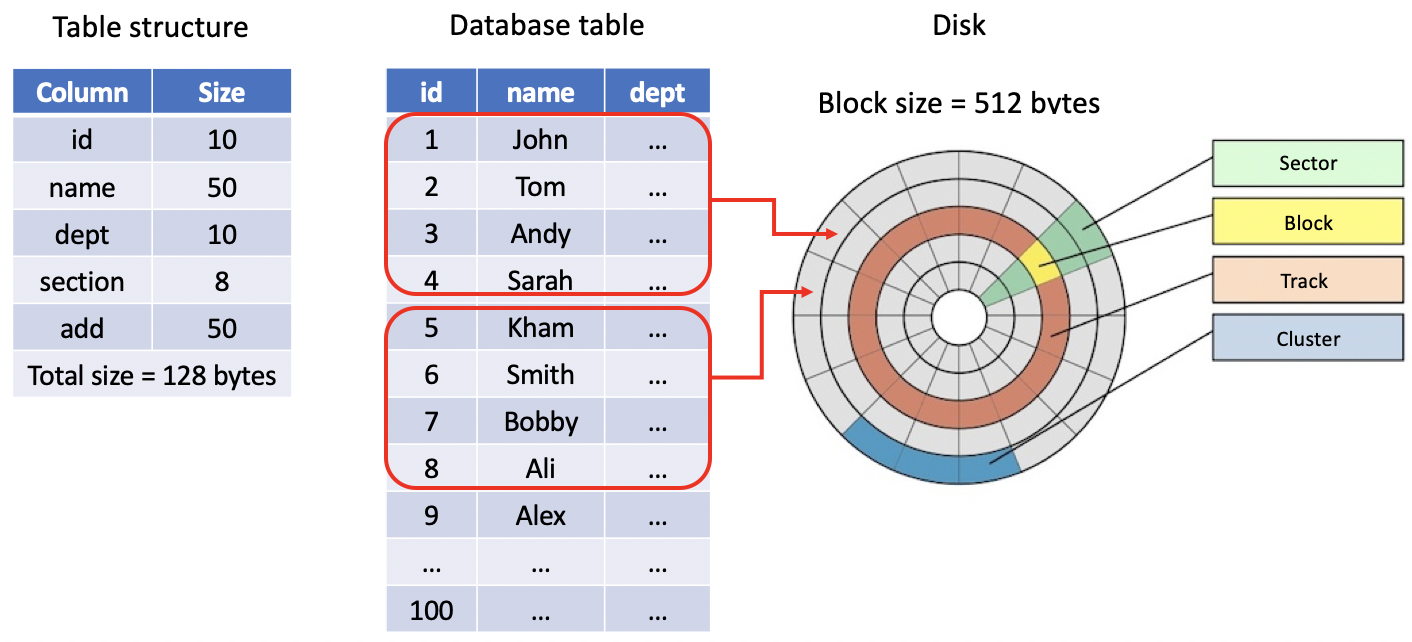$\text{number of records/block} = \dfrac{512}{128}=4$

$\text{number of blocks for database table} = \dfrac{100}{4}=25$

## 4. 索引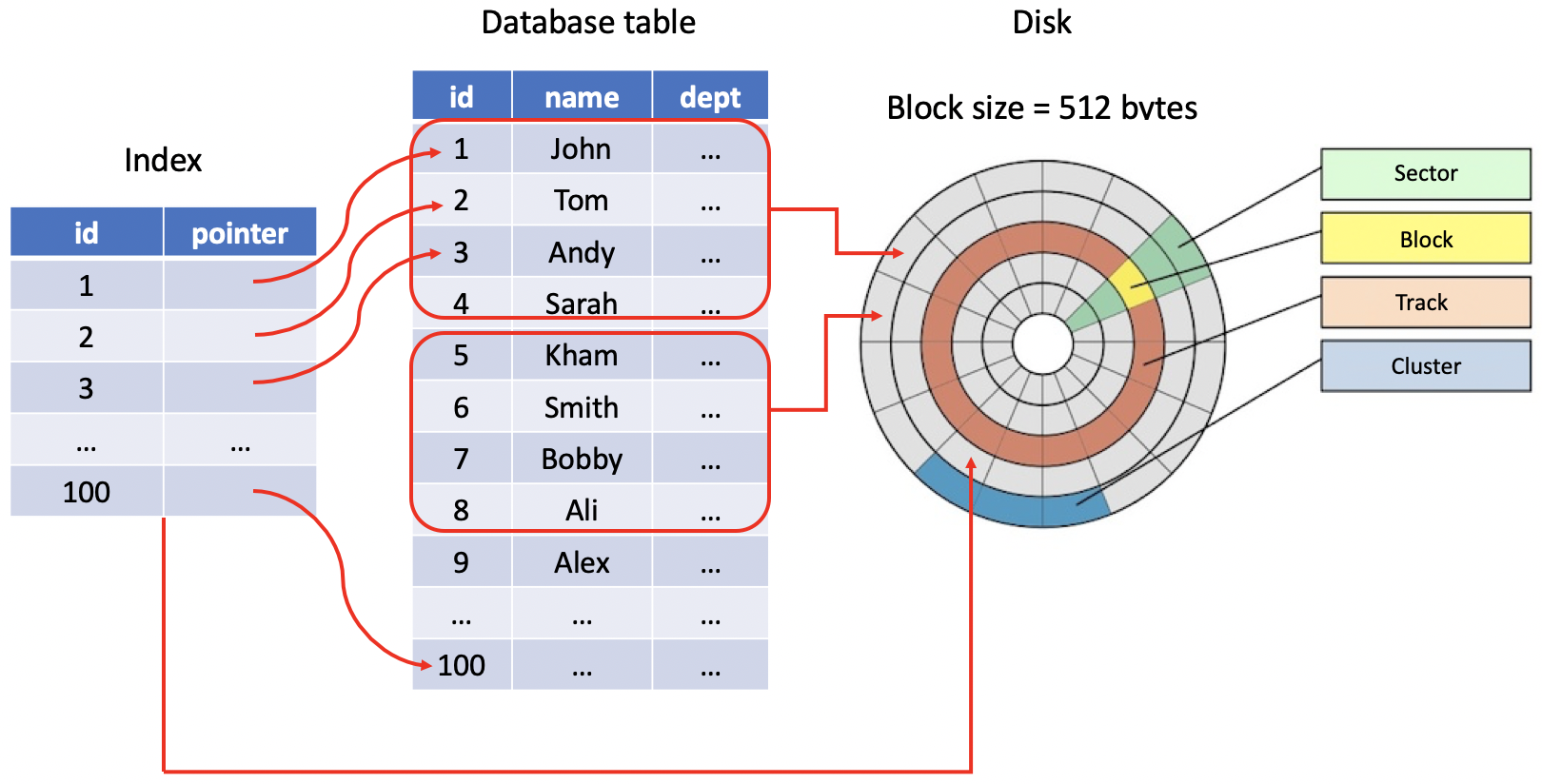$\text{number of entries/block} = \dfrac{512}{16}=32$

$\text{number of blocks for index} = \dfrac{100}{32}=3.125$

## 5. 多级索引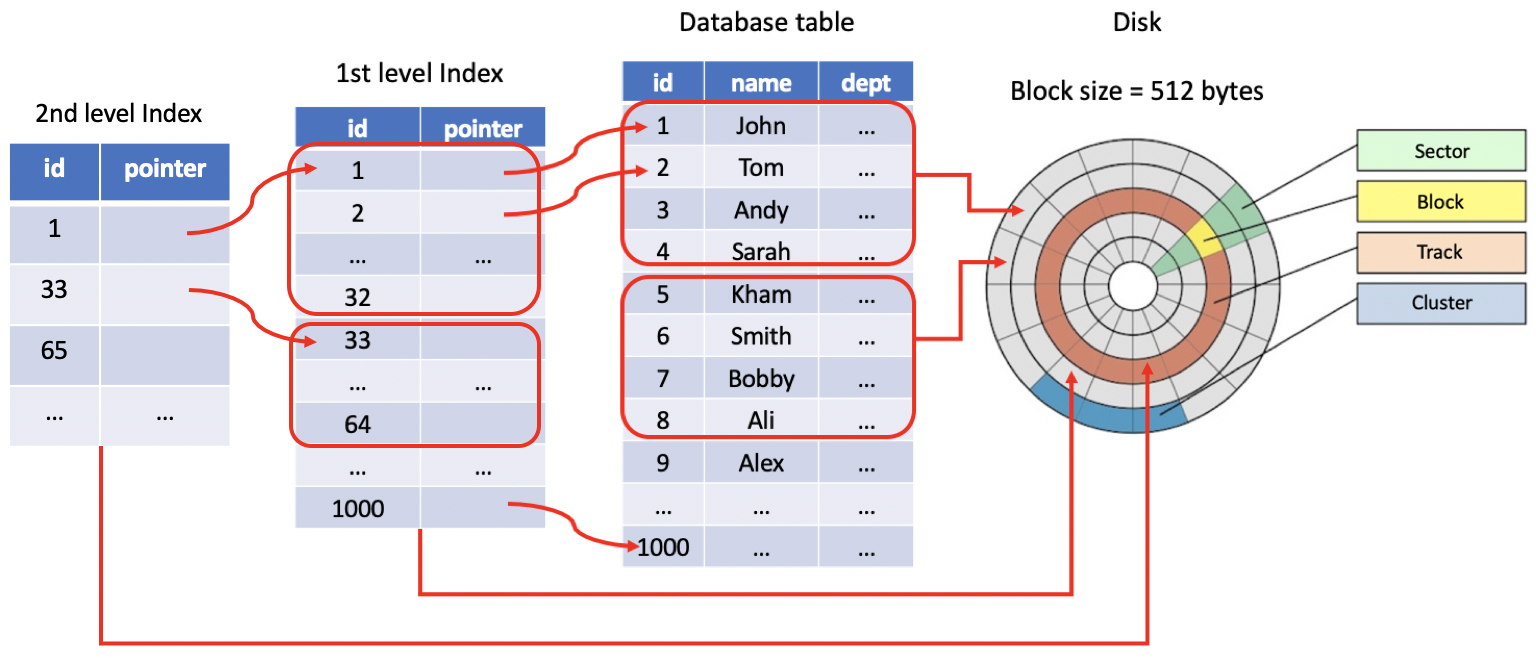$\text{number of entries for 2nd index} = \dfrac{1000}{32}=31.25$

$\text{number of blocks for 2nd index} = \dfrac{32}{32}=1$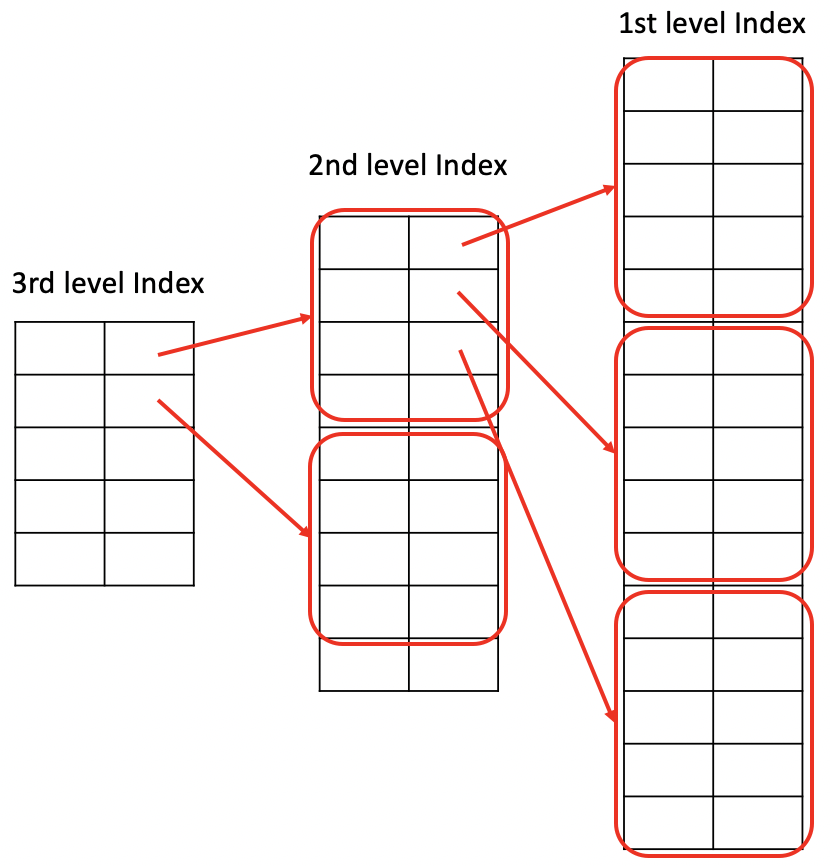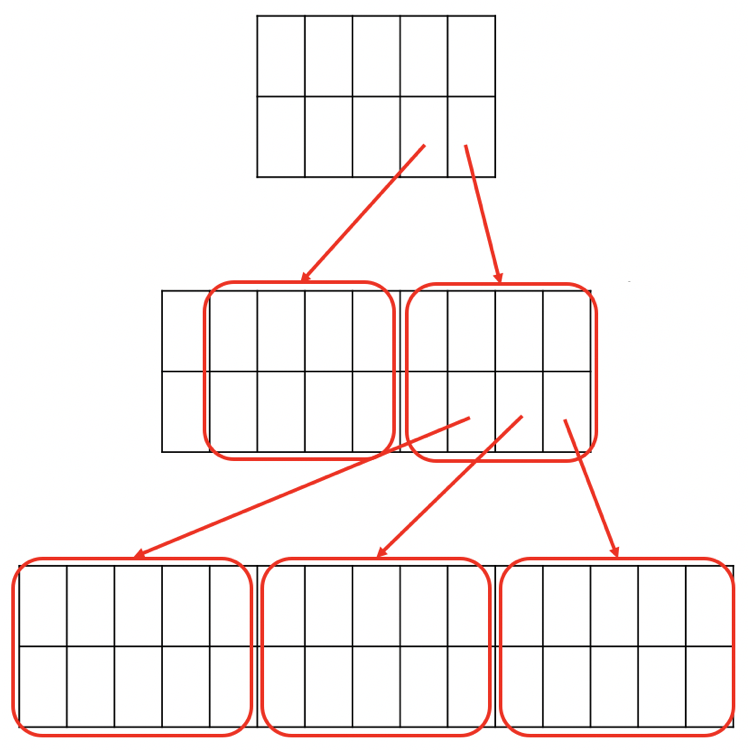## 6. 多路查找树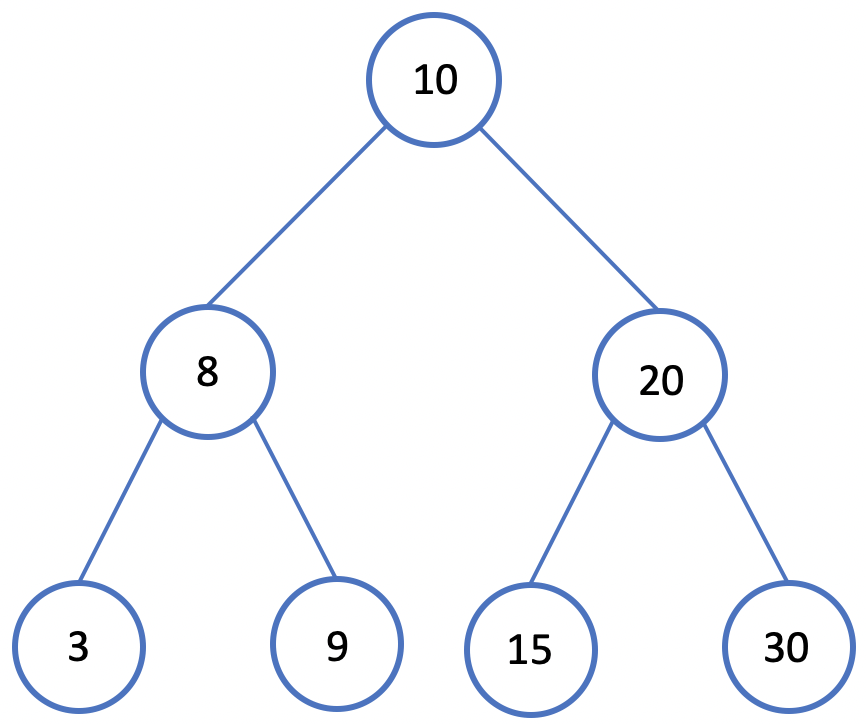1. 若任意结点的左子树不空，则左子树上所有结点的值均小于它的根结点的值；
2. 若任意结点的右子树不空，则右子树上所有结点的值均大于或等于它的根结点的值；
3. 任意结点的左、右子树也分别为二叉查找树。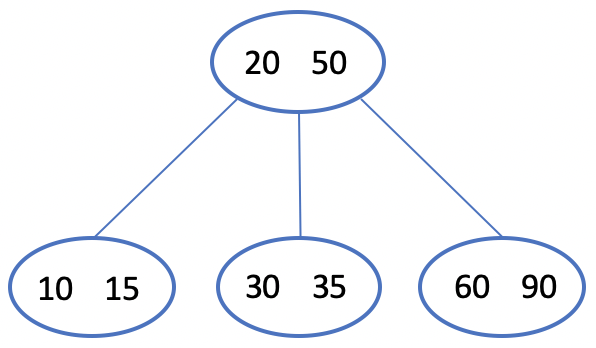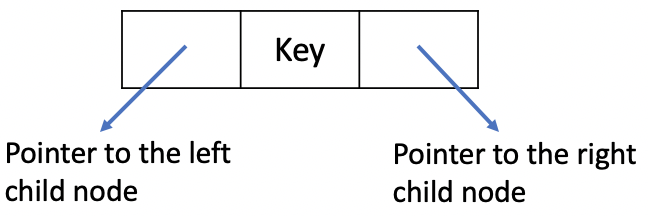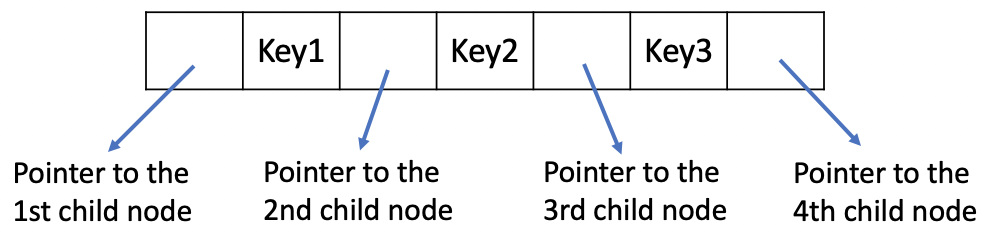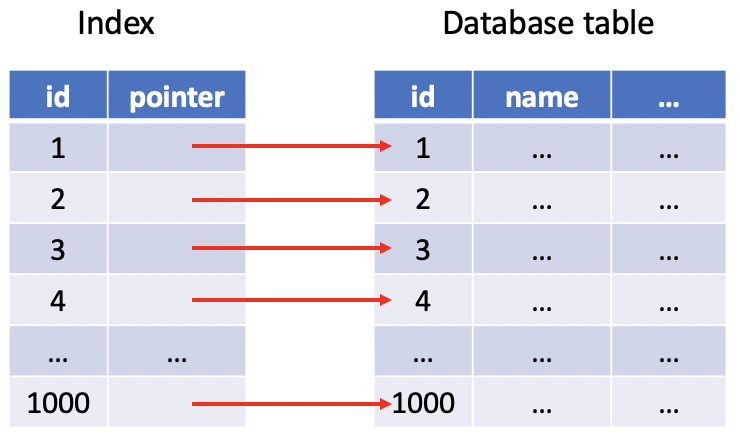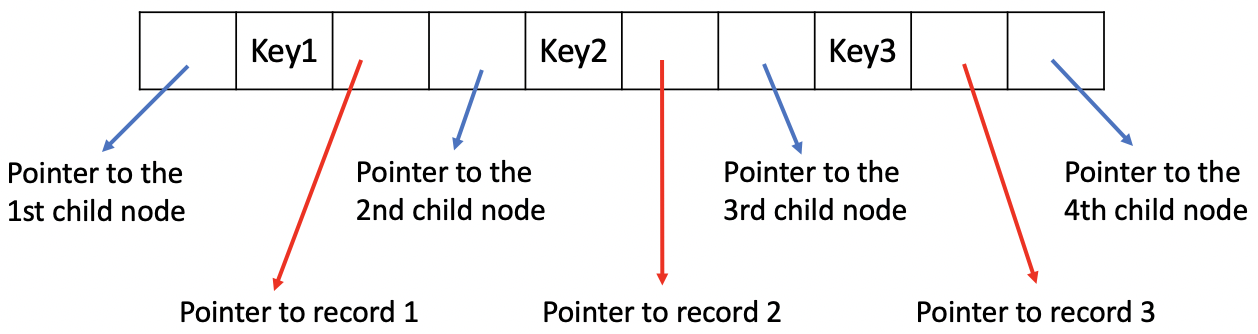Keys: $10, 20, 30$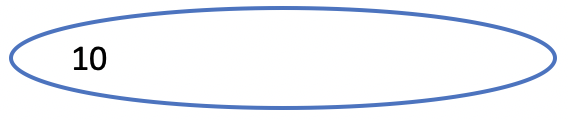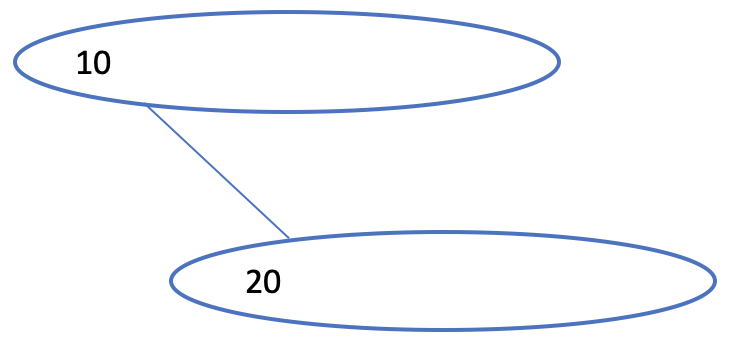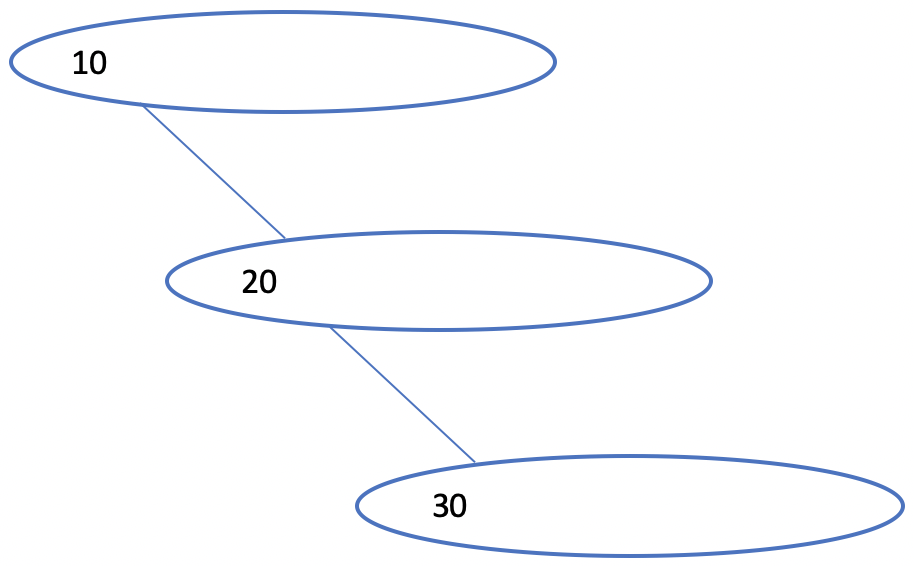## 7. B 树

1. 每个结点必须填满至少一半的 key。对于 M 路查找树，每个结点要至少包含 $\lceil m/2 \rceil$ 个 key。例如，对于 $10$ 路查找树，每个结点至少需要包含 $5$ 个 key，然后我们才会考虑创建新的结点。这条规则可以帮助我们控制树的高度。
2. 根结点可以有至少 $2$ 个子结点
3. 所有的叶子结点必须在同一层
4. 树的创建过程是由底向上的

• $M=4$
• Keys: $10, 20, 40, 50$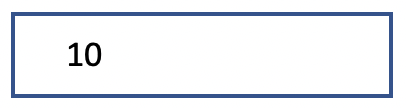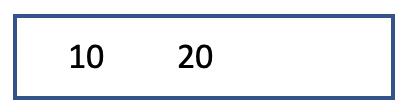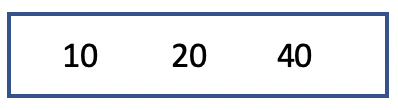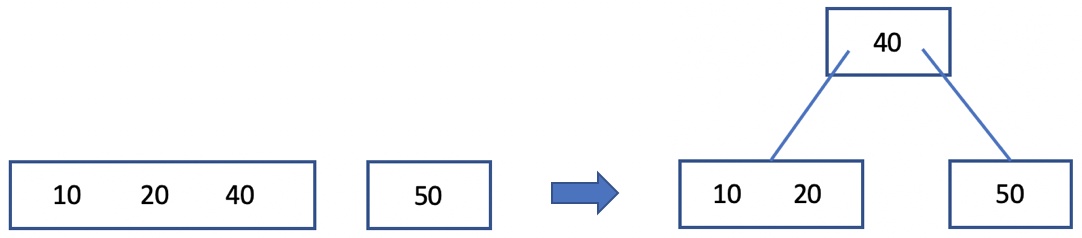B 树非常适合用于实现多级索引结构，它可以根据 key 的数量自动构建高层级的索引。对于上面的例子，我们可以继续插入一些新的 key，来观察 B 树是如何自动构建多级索引的。

Keys: $10, 20, 40, 50, 60 ,70, 80, 30, 35, 5, 15$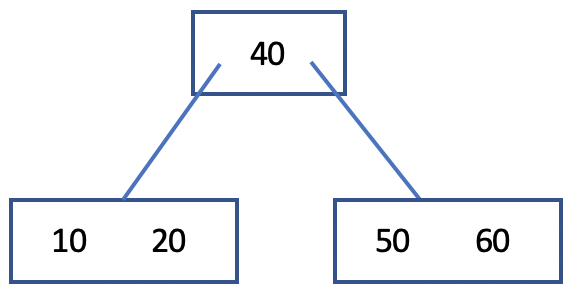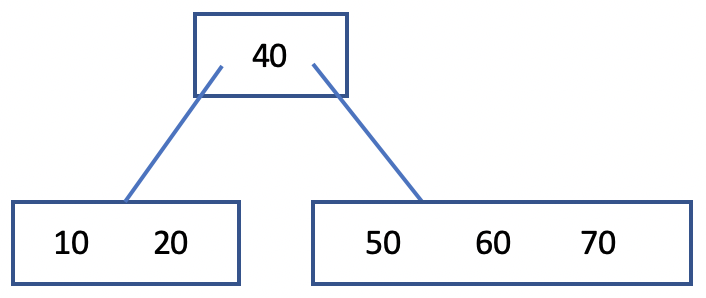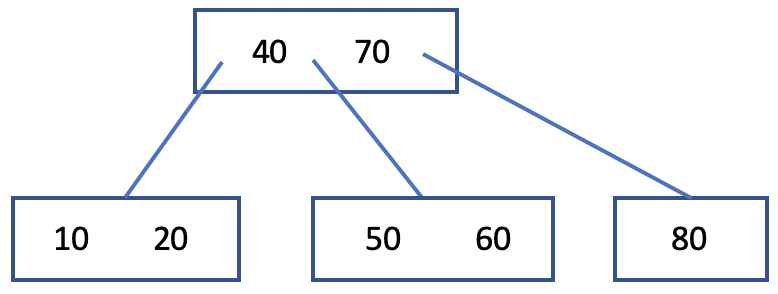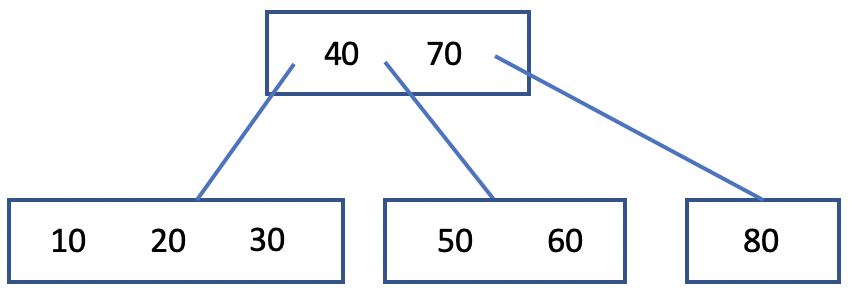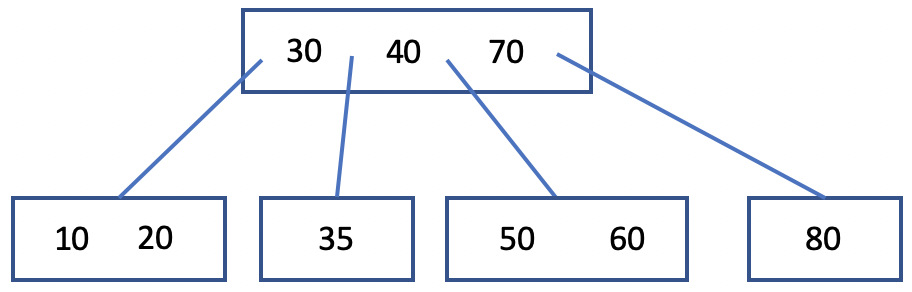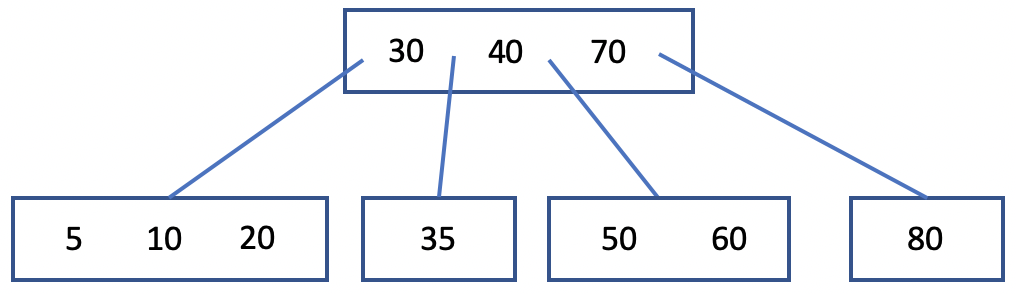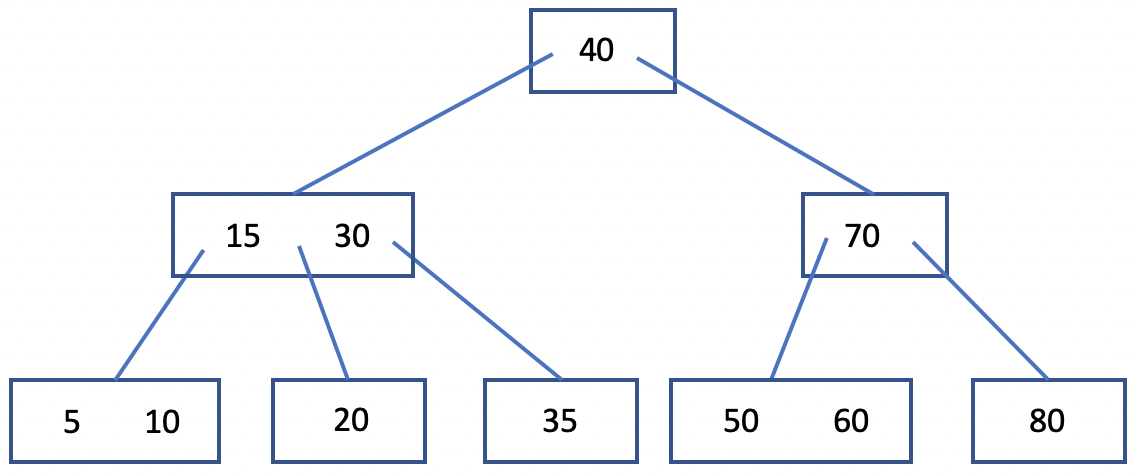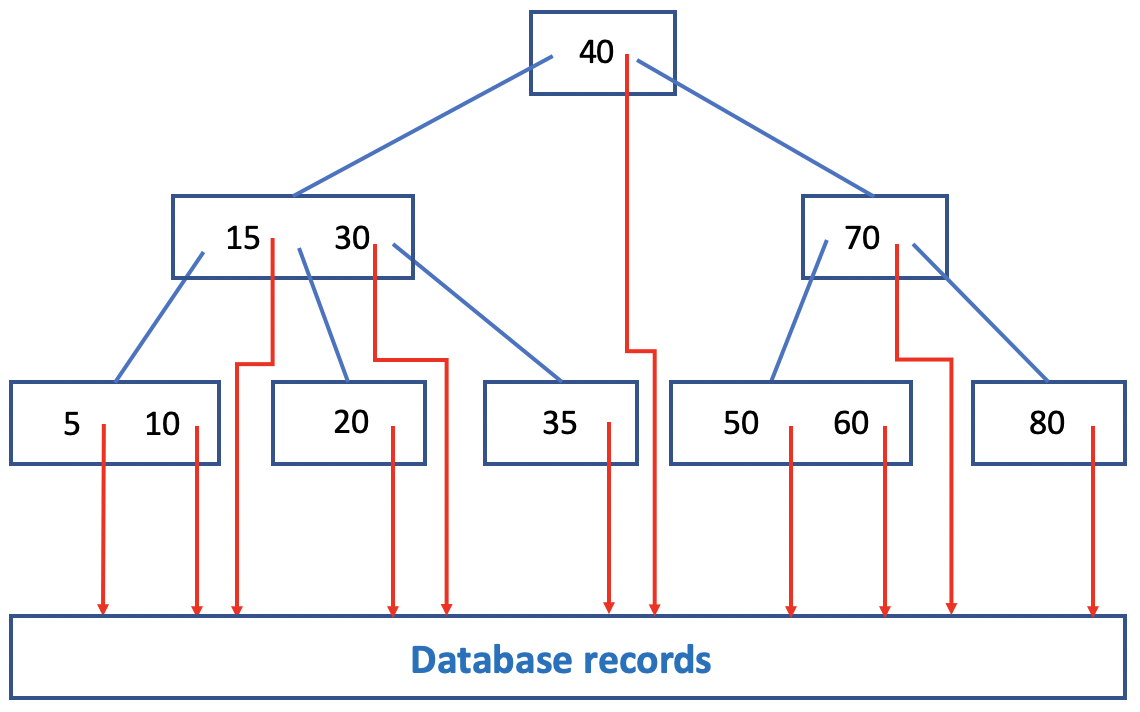## 8. B+ 树B+ 树的这种结构与我们之前讨论的多级索引结构完全一样。B+ 树与 B 树的主要区别在于：

1. B+ 树中，叶子结点中保存了所有的 key，其中有些 key 在非叶子结点中也进行了冗余存储。
2. B+ 树中，非叶子结点不再包含记录指针，所有的记录指针都保存在叶子结点中。
3. B+ 树中，所有的叶子结点构成了一个链表。因此，叶子结点所在的层实际上构成了一层密集索引，而非叶子结点所在的层构成了稀疏索引。

## 9. 总结本作品采用知识共享署名-非商业性使用-相同方式共享 4.0 国际许可协议进行许可。 欢迎转载，并请注明来自：YEY 的博客 同时保持文章内容的完整和以上声明信息！